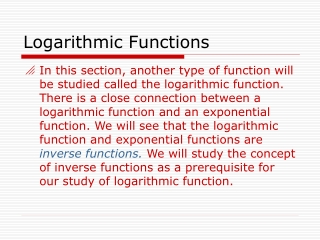DownloadDownload PresentationLogarithmic Functions

# Logarithmic Functions

Télécharger la présentation## Logarithmic Functions

- - - - - - - - - - - - - - - - - - - - - - - - - - - E N D - - - - - - - - - - - - - - - - - - - - - - - - - - -
##### Presentation Transcript

1. Logarithmic Functions • In this section, another type of function will be studied called the logarithmic function. There is a close connection between a logarithmic function and an exponential function. We will see that the logarithmic function and exponential functions are inverse functions. We will study the concept of inverse functions as a prerequisite for our study of logarithmic function.

2. One to one functions We wish to define an inverse of a function. Before we do so, it is necessary to discuss the topic of one to one functions. First of all, only certain functions are one to one. Definition: A function is said to be one to one if distinct inputs of a function correspond to distinct outputs. That is, if

3. Graph of one to one function • This is the graph of a one to one function. Notice that if we choose two different x values, the corresponding values are also different. Here, we see that if x =- 2 , y = 1 and if x = 1, y is about 3.8. • Now, choose any other • pair of x values. Do you • see that the • corresponding y • values will always be different?

4. Horizontal Line Test • Recall that for an equation to be a function, its graph must pass the vertical line test. That is, a vertical line that sweeps across the graph of a function from left to right will intersect the graph only once. • There is a similar geometric test to determine if a function is one to one. It is called the horizontal line test. Any horizontal line drawn through the graph of a one to one function will cross the graph only once. If a horizontal line crosses a graph more than once, then the function that is graphed is not one to one.

5. Which functions are one to one?

6. Definition of inverse function • Given a one to one function, the inverse function is found by interchanging the x and y values of the original function. That is to say, if ordered pair (a,b) belongs to the original function then the ordered pair (b,a) belongs to the inverse function. Note: If a function is not one to one (fails the horizontal line test) then the inverse of such a function does not exist.

7. Logarithmic Functions • The logarithmic function with base two is defined to be the inverse of the one to one exponential function • Notice that the exponential • function • is one to one and therefore has an inverse.

8. Inverse of exponential function • Start with • Now, interchange x and y coordinates: • There are no algebraic techniques that can be used to solve for y, so we simply call this function y the logarithmic function with base 2. • So the definition of this new function is • if and only if • (Notice the direction of the arrows to help you remember the formula)

9. Graph, domain, range of logarithmic function • 1. The domain of the logarithmic function is the same as the range of the exponential function • (Why?) • 2. The range of the logarithmic function is the same as the domain of the exponential function • (Again, why?) • 3. Another fact: If one graphs any one to one function and its inverse on the same grid, the two graphs will always be symmetric with respect the line y = x.

10. Three graphs: , , y = x Notice the symmetry:

11. Logarithmic-exponential conversions • Study the examples below. You should be able to convert a logarithmic into an exponential expression and vice versa. • 1. • 2. • 3. • 4.

12. Solving equations Using the definition of a logarithm, you can solve equations involving logarithms: See examples below:

13. Properties of logarithms • These are the properties of logarithms. M and N are positive real numbers, b not equal to 1, and p and x are real numbers.

14. Solving logarithmic equations • Solve for x: • Product rule • Special product • Definition of log • X can be 10 only • Why?

15. Another example • Solve: • 2. Quotient rule • 3. Simplify • (divide out common factor of pi) • 4. rewrite • 5 definition of logarithm • 6. Property of exponentials

16. Common log Natural log Common logs and Natural logs

17. Solve for x. Obtain the exact solution of this equation in terms of e (2.71828…) Quotient property of logs Definition of (natural log) Multiply both sides by x Collect x terms on left side Factor out common factor Solve for x Solution: Solving an equation

18. Solve the equation Take natural logarithm of both sides Exponent property of logarithms Distributive property Isolate x term on left side Solve for x Solution: Solving an exponential equation

19. How long will it take money to double if compounded monthly at 4 % interest ? 1. compound interest formula 2. Replace A by 2P (double the amount) 3. Substitute values for r and m 4. Take ln of both sides 5. Property of logarithms 6. Solve for t and evaluate expression Solution: Application